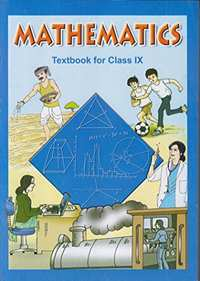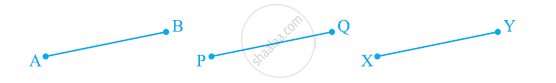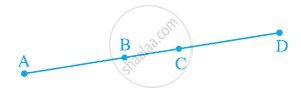Share

Books Shortlist

NCERT solutions for Class 9 Mathematics chapter 5 - Introduction to Euclid's Geometry

Mathematics Textbook for Class 9

NCERT Mathematics Class 9Chapter 5: Introduction to Euclid's Geometry

Ex. 5.10Ex. 5.20

Chapter 5: Introduction to Euclid's Geometry Exercise 5.10 solutions [Pages 85 - 86]

Ex. 5.10 | Q 1 | Page 85

Which of the following statements are true and which are false? Give reasons for your answers.

(i) Only one line can pass through a single point.

(ii) There are an infinite number of lines which pass through two distinct points.

(iii) A terminated line can be produced indefinitely on both the sides.

(iv) If two circles are equal, then their radii are equal.

(v) In the following figure, if AB = PQ and PQ = XY, then AB = XYEx. 5.10 | Q 2.1 | Page 85

Give a definition for parallel lines. Are there other terms that need to be defined first? What are they, and how might you define them?

Ex. 5.10 | Q 2.2 | Page 85

Give a definition for perpendicular lines. Are there other terms that need to be defined first? What are they, and how might you define them?

Ex. 5.10 | Q 2.3 | Page 85

Give a definition for line segment. Are there other terms that need to be defined first? What are they, and how might you define them?

Ex. 5.10 | Q 2.4 | Page 85

Give a definition for radius of a circle. Are there other terms that need to be defined first? What are they, and how might you define them?

Ex. 5.10 | Q 2.5 | Page 85

Give a definition for square. Are there other terms that need to be defined first? What are they, and how might you define them?

Ex. 5.10 | Q 3 | Page 85

Consider two ‘postulates’ given below:-

(i) Given any two distinct points A and B, there exists a third point C which is in between A and B.

(ii) There exist at least three points that are not on the same line.

Do these postulates contain any undefined terms? Are these postulates consistent? Do they follow from Euclid’s postulates? Explain.

Ex. 5.10 | Q 4 | Page 86

If a point C lies between two points A and B such that AC = BC, then prove that Ac =  1/2AB. Explain by drawing the figure.

Ex. 5.10 | Q 5 | Page 86

If a point C lies between two points A and B such that AC = BC, point C is called a mid-point of line segment AB. Prove that every line segment has one and only one mid-point.

Ex. 5.10 | Q 6 | Page 86

In the following figure, if AC = BD, then prove that AB = CD.Ex. 5.10 | Q 7 | Page 86

Why is Axiom 5, in the list of Euclid’s axioms, considered a ‘universal truth’? (Note that the question is not about the fifth postulate.)

Chapter 5: Introduction to Euclid's Geometry Exercise 5.20 solutions [Page 88]

Ex. 5.20 | Q 1 | Page 88

How would you rewrite Euclid’s fifth postulate so that it would be easier to understand?

Ex. 5.20 | Q 2 | Page 88

Does Euclid’s fifth postulate imply the existence of parallel lines? Explain.

Chapter 5: Introduction to Euclid's Geometry

Ex. 5.10Ex. 5.20

NCERT Mathematics Class 9NCERT solutions for Class 9 Mathematics chapter 5 - Introduction to Euclid's Geometry

NCERT solutions for Class 9 Maths chapter 5 (Introduction to Euclid's Geometry) include all questions with solution and detail explanation. This will clear students doubts about any question and improve application skills while preparing for board exams. The detailed, step-by-step solutions will help you understand the concepts better and clear your confusions, if any. Shaalaa.com has the CBSE Mathematics Textbook for Class 9 solutions in a manner that help students grasp basic concepts better and faster.

Further, we at shaalaa.com are providing such solutions so that students can prepare for written exams. NCERT textbook solutions can be a core help for self-study and acts as a perfect self-help guidance for students.

Concepts covered in Class 9 Mathematics chapter 5 Introduction to Euclid's Geometry are Equivalent Versions of Euclid’S Fifth Postulate, Euclid’S Definitions, Axioms and Postulates, Concept for Euclid’S Geometry.

Using NCERT Class 9 solutions Introduction to Euclid's Geometry exercise by students are an easy way to prepare for the exams, as they involve solutions arranged chapter-wise also page wise. The questions involved in NCERT Solutions are important questions that can be asked in the final exam. Maximum students of CBSE Class 9 prefer NCERT Textbook Solutions to score more in exam.

Get the free view of chapter 5 Introduction to Euclid's Geometry Class 9 extra questions for Maths and can use shaalaa.com to keep it handy for your exam preparation

S